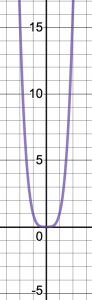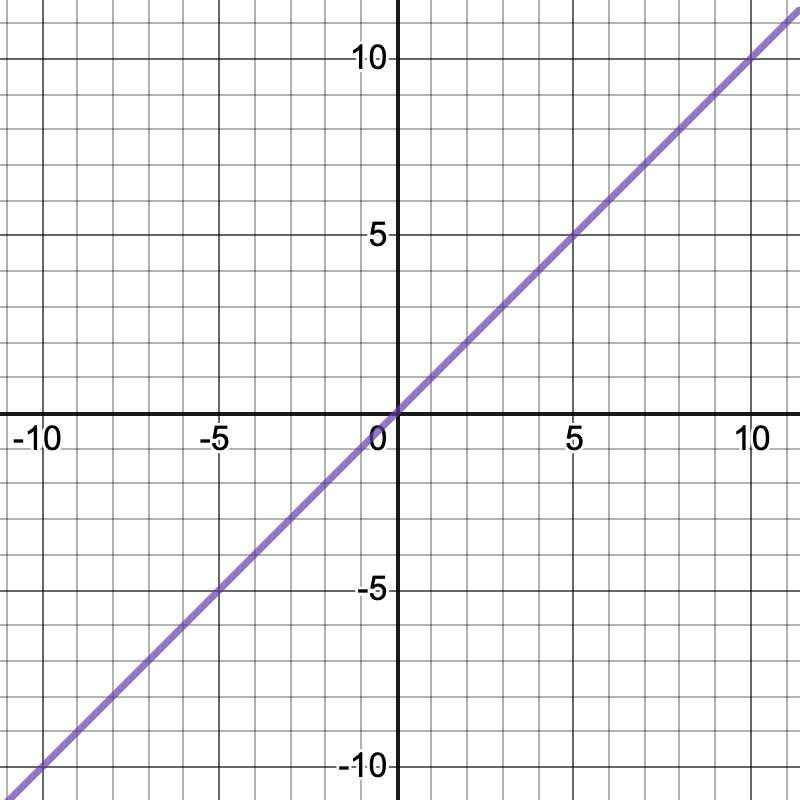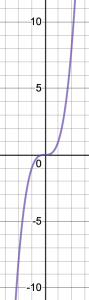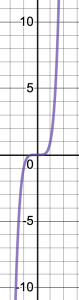## End Behavior, Degree, and Leading Coefficient

This post about end behavior, degree, and leading coefficient of a polynomial function is part of a series of posts to help you prepare for the Advanced Algebra and Functions part of the Accuplacer test.

## Question

Consider the function

where a and c are integers and are constants and c is positive. The the graph y = g(x) goes toward negative infinity at both negative and positive extremes of x. Is positive or negative? Is c even or odd?

## Solution

Let’s deal with c first.

### Sidebar: even and odd polynomial functions

The end behavior of a polynomial function with an even degree is in the same direction — either toward negative infinity or toward positive infinity — for both extremes of x. Think, for example, of  . It goes up toward positive infinity as the absolute value of x increases, whether positive or negative. So do , , etc.If you multiply any of those expressions by a leading coefficient of -1, or any negative number, then end behavior goes to negative infinity for both extremely negative and extremely positive values of x.

On the other hand, the end behavior of a polynomial with an odd degree is in opposite directions for extremely negative and extremely positive values of x. Think of , , etc.So far, I’ve been talking about a monomial —  or  or y equals x to some other power. What happens in the more general case, when you get a polynomial with several terms, for example ?When a polynomial contains terms of lower degree than the leading term, you get some noise, but end behavior is still determined by the highest-degree term. Note that as x gets very negative or very positive, the graph of  starts to look more like the graph of .

### Back to the Question

The function in question, , has degree c + 1: The c comes from the power c on the term x + 4, and the +1 comes from the implied power of 1 on the term x – 3, for a total power of x  equal to c + 1. Thus c + 1 is the degree of function g. And you’re told that this function’s end behavior is to approach negative infinity at both extremes of x. As shown above, a polynomial that goes in the same direction — either up or down — for both extremely positive and extremely negatives values of x has an even degree. That means c+1 is even, so c is odd.

What about a? As shown above, a polynomial with even degree, like this one, that goes down at both extremes of x starts with a negative number. So a is negative.

This question is similar to question number 17 in the sample questions for the Accuplacer Advanced Algebra and Functions test.

## Finding a Function’s Range

This post about finding a function’s range from its equation is part of a series of posts to help you prepare for the Advanced Algebra and Functions part of the Accuplacer test.

## Question

Find the range of this function:

## Solution

A function’s range is the set of values y can take in that function.

You are asked to find a function’s range. What are the values y can take in this function?

Consider the first term, . Any real number (unless otherwise stated, it’s safe to assume x is real) to an even power, like 4, has a positive value or zero. It just can’t be negative. The lowest value of is zero — that happens where  — and there is no limit to how high can go, so  ranges from 0 to infinity. Then its negative,  ranges from negative infinity to 0.The second term, 5. increases each y-value by 5. At the low end of the range, negative infinity plus 5 is still negative infinity, so the bottom of the range is still negative infinity. At the top end, , so the top of the range is 5.

So the range of is from negative infinity to 5. In other words, . In interval notation, that’s .

This question is similar to question number 11 in the sample questions for the Accuplacer Advanced Algebra and Functions test.

## Rationalizing a Denominator

This post about rationalizing a denominator is part of a series of posts to help you prepare for the Advanced Algebra and Functions part of the Accuplacer test.

## Question

Consider the expression

where a and b are positive integers.

Which of the following expressions is equivalent to it?

A.

B.

C.

D.

## Solution

Only one of the four answer choices is a fraction. See if you can convert the expression that you are given into a format that is not a fraction and perhaps get one of the answer choices. Is there a way to make that denominator go away?

### Sidebar: Rationalizing a denominator using a conjugate

A denominator that contains radicals is considered poor form. You may sometimes be asked to rationalize such denominators, meaning convert the fraction or rational expression into a format that has no radicals in its denominator. The usual way to do that is to use the denominator’s conjugate. The conjugate of an expression is .

For , multiply and divide by the denominator’s conjugate to rationalize the denominator, which is likely a step in the right direction. (To multiply an expression by something and divide by the same thing changes the expression’s form but not its value.) Let’s try that.

The conjugate of

is

Multiplying and dividing by the conjugate looks like:

All the radicals have dropped out of the denominator. That’s one thing we were looking for. Still, we can simplify: Notice that  is a factor in both the numerator and the denominator. Cancel it and you get

This is answer choice C. So you’re done.

For more about rational numbers, expressions, and equations, see Solving a Rational Equation

The Accuplacer sample problem on which this one is based is #19 in the sample questions for the Accuplacer Advanced Algebra and Functions test.

## Solving a Rational Equation

This post about solving a rational equation is #15 in a series of posts to help you prepare for the Advanced Algebra and Functions part of the Accuplacer test.

## Question

Solve this rational equation:

Assume x is not equal to -8 or 2/3.

## Solution

What do we mean by rational? The root is ratio. A rational number is a number that can be expressed as quotient — a ratio — of two integers. A rational expression is a quotient — a ratio — of algebraic expressions. A rational equation is an equation comprised of rational expressions.

To get started solving this rational equation, multiply both sides by . That should give you:

Cancel the terms that show up in both numerator and denominator. That’s on the left and on the right. That leaves:

(Yes, I could have just said “cross multiply,” but I hate that expression. It’s a personal quirk.) Now we’re done with denominators.

Distribute:

Subtract  from both sides, and then switch the sides.

Factor:

Set each factor equal to 0:

### Caution

Remember that division by zero yields something undefined, so an x-value that makes a denominator zero is not a valid answer. So you need to make sure that the x values you’ve found do not make one of the denominators in the original question zero. To find those values, set those denominators equal to zero:

Oh, look: Those are the values that the question says are excluded. The question is telling you that a solution that causes the denominator to be zero is not a valid solution. But today that is not your problem, because that’s not one of the answers we found. We found and . Interesting factoid: The official practice question on which this question is based stipulates that x is not equal to -3/2 and then offers -3/2 as an answer choice. If there’s a lesson in that, it may be that it’s a good idea to reread the question before you give your final answer.

To complete the check, substitute  and  into the original equation.

This question is similar to question number 15 in the sample questions for the Accuplacer Advanced Algebra and Functions test.